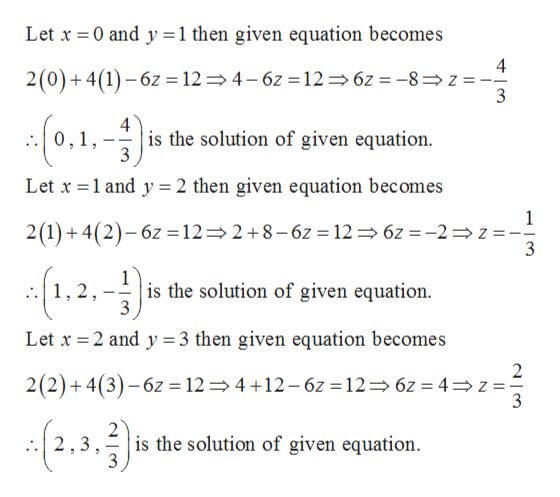# For Exercise, find three ordered triples that are solutions to the linear equation in three variables.2x + 4y − 6z = 12

Question
1 views

For Exercise, find three ordered triples that are solutions to the linear equation in three variables.

2x + 4y − 6z = 12

check_circle

Step 1

Given,

Step 2

To find the ordered triplets that are solutions to given equation, we choose some...help_outlineImage TranscriptioncloseLet x = 0 and y =1 then given equation becomes 2(0)+ 4(1) – 6z = 12= 4– 6z = 12=6z = -8= z = (0.1.-) is the solution of given equation. ,1, 3 Let x =1 and y = 2 then given equation becomes 2(1)+ 4(2)– 6z =12=2+8-6z = 12= 6z =-2=z =-. {1.2.) is the solution of given equation. 3 .1, 2, Let x = 2 and y =3 then given equation becomes 2(2)+ 4(3)– 6z = 12= 4 +12- 6z =12= 6z = 4=z= 3 (2,.3.) is the solution of given equation. 4- 3. fullscreen

### Want to see the full answer?

See Solution

#### Want to see this answer and more?

Solutions are written by subject experts who are available 24/7. Questions are typically answered within 1 hour.*

See Solution
*Response times may vary by subject and question.
Tagged in

### Equations and In-equations